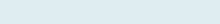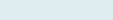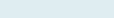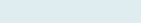# Average last Nth Numbers

When you want to calculate the average of the last four, five or any nth values in the columns or rows with the help of formula offset and count.

Query: We have the name of the student with marks of each test. Our task is to get the average marks of test 5 to test 9 (C7: C11) of Joe. Same find for all students.

Formula to implement:

=AVERAGE(OFFSET(D3,COUNT(D3:D11)-5,0,5))

Note: You can simply add the Excel filter to average only the Lowest and Largest Nth value in a range with this formula.

Functions applicable:

Average: Get an average of numbers positioned in one or multiple ranges.

Offset: The Excel OFFSET function returns one or multiple data points of a data set by providing a reference point of data set with a specified number of rows and columns to offset.

Count: The Excel COUNT function compute the count of numbers within the reference list of arguments ignoring the text and blank cells.

Formula Explanation (step by step)

Excel starts calculating innermost parenthesis than work outward. You can also debug the formula using a shortcut F9 function key under the formulas tab.

Let’s break the formula into multiple small steps to understand its application.

First formula:

COUNT(C3:C11)

Syntax:  COUNT(value1, [value2], …)

Step 1:Result: 9

It will give count of (C3:C11) i.e. 9.

Second formula:

OFFSET(C3,COUNT(C3:C11)-5,0,5)

Syntax: OFFSET(reference, rows, cols, [height], [width])

Step 2:Result: C7:C11

After giving count (9), OFFSET function decrease the range by 5 so new starting point is C7 from C3 with height are of 5 (C7:C11). The last 5 numbers.

Third formula:

AVERAGE(OFFSET(C3,COUNT(C3:C11)-5,0,5))

Syntax: AVERAGE(number1, [number2], …)

Step 3:Result: 30.4

77+12+0+40+23/5= 152/5= 30.4

It will give the average of last five numbers in the given range C7:C11(77+12+0+40+23).

Note: If you want to find the average of the highest values in the array.